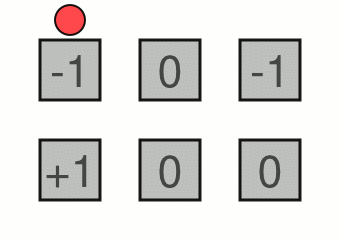Your browser (Unknown 0) is no longer supported. Some parts of the website may not work correctly. Please update your browser.

#### BallSwitchBoard

Calculate how balls roll through a board of switches.

A board consisting of ball switches arranged into N rows and M columns is given. A ball switch is a device that can change the direction of a rolling ball. It has the following properties:

• it is square-shaped;
• it can be in one of three modes: −1, 0 or +1;
• a ball can enter it through the top or the left edge;
• a ball can leave it through the bottom or the right edge;
• if the mode is −1, a ball entering the device will leave it through the bottom edge;
• if the mode is +1, a ball entering the device will leave it through the right edge;
• if the mode is 0, a ball entering the device will not change direction;
• after a ball leaves the device, its mode is immediately negated.

K balls are rolled through the board one by one. Each ball enters the board through the top edge of the top-left ball switch. Then it rolls through the board and possibly changes its direction depending on the modes of the switches through which it passes. Eventually it leaves the board either:

• through the bottom edge of one of the switches in the bottom row; or
• through the right edge of one of the switches in the rightmost column.

For example, consider a board consisting of 2 rows and 3 columns. The following image shows the initial modes of all switches and how the first four balls will roll through the board.Write a function:

class Solution { public int solution(int[][] A, int K); }

that,given a matrix A consisting of N rows and M columns describing a board of ball switches and a non-negative number K of balls inserted into this board, returns the number of balls that will leave the board through the bottom edge of the bottom-right switch.

The matrix A describes the board as follows:

• each element of the matrix has value −1, 0 or +1 and describes the initial mode of the corresponding ball switch;
• element A corresponds to the top-left ball switch;
• element A[N−1][M−1] corresponds to the bottom-right ball switch.

For example, given K = 4 and matrix A such that:

A = -1 A = 0 A = -1 A = 1 A = 0 A = 0

the function should return 1, because:

• the balls roll through the board as shown in the image above;
• only the second ball leaves the board through the bottom edge of the bottom-right ball switch.

Write an efficient algorithm for the following assumptions:

• N and M are integers within the range [1..1,000];
• each element of matrix A is an integer that can have one of the following values: −1, 0, 1;
• K is an integer within the range [0..1,000,000,000];
• the matrix A correctly describes some board of ball switches;
• balls are inserted in to the board and roll through it according to the rules specified above.